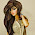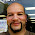## Friday, February 1, 2013

### Balances and Recursion Formula - Part 1Greetings everyone coming to you from a Starbucks in Covina, CA! (on my way to seeing my mom and some friends for dinner).

1 month of 2013 is already in the books - hope everyone is getting February off to a great start!

The topic of this and the next post: using a recursion formula in financing.

The scenario: You have a loan that you make monthly payments. Interest accrues each month based on the loan's balance. The rate is stated as an annual rate. How many months will it take to pay off the loan?

For this, we are going to use a recursion formula to describe the balance at the end of each payment. We will then come up with a general formula which will allow us to find the balance at any month. Finally, use the general formula to find when the balance is zero (approximately).

Part 1 will deal with the scenario stated. On my next blog entry, I will consider paying the loan balance when a monthly charge is involved.

What is a recursion formula?

A recursion formula is a formula in which the result is based on previous results. To generate a sequence of answers, one or more initial conditions are required.

A simple example is:

a_(n+1) = 2 × a_n + 1 with the initial condition a_0 = 0.

In order to calculate a_(n+1), you will need the previous result, a_n.

Then: (a_n is in bold)

a_0 = 0 (start with the initial condition)
a_1 = 2 × 0 + 1 = 1
a_2 = 2 × 1 + 1 = 3
a_3 = 2 × 3 + 1 = 7
a_4 = 2 × 7 + 1 = 15
And so on...

Some recursion formulas can be transformed into general formulas, where any nth term can be found when only the initial conditions are known.

A famous recursion formula is the Fibonacci Sequence (1, 1, 2, 3, 5, 8, 13, 21, etc...)

a_(n+2) = a_(n+1) + a_n with the initial conditions a_0 = 1 and a_1 =1.

Back to our scenario: You have a loan that you make monthly payments. Interest accrues each month based on the loan's balance. The rate is stated as an annual rate. How many months will it take to pay off the loan?

Variables:
b = a_0 = initial balance
p = monthly payment
r = annual rate (if the rate is 10%, r = 10)
a_n = balance after n payments (after n months)

Let the recursion formula be:
a_(n+1) = a_n + a_n × r/1200 - p
with the initial condition a_0 = b

Let θ = r/1200. Then:

a_(n+1) = a_n × (1 + θ) - p

Note: we will stop when the balance sinks below the payment amount. At that point, the next payment is the balance, reducing the debt to 0.

Calculating the first few terms (balance after n payments) in terms of the initial balance (a_0):

a_1 = a_0 × (1 + θ) - p

a_2 = a_1 × (1 + θ) - p
a_2 = (a_0 × (1 + θ) - p) - p
a_2 = a_0 × (1 + θ)^2 - p × (1 + θ) - p

a_3 = a_2 × (1 + θ) - p
a_3 = (a_0 × (1 + θ)^2 - p × (1 + θ) - p) × (1 + θ) - p
a_3 = a_0 × (1 + θ)^3 - p × (1 + θ)^2 - p × (1 + θ) - p

Noticing the pattern...

a_n = a_0 × (1 + θ)^n - Σ( p × (1 + θ)^k, k=0, n-1)
a_n = a_0 × (1 + θ)^n - p × Σ( (1 + θ)^k, k=0, n-1)
a_n = a_0 × (1 + θ)^n - p × ((1 + θ)^n - 1)/(1 + θ - 1))

With a_0 = b:

a_n = b × (1 + θ)^n - p × ((1 + θ)^n - 1)/θ

Example 1:

A bank loan is made for \$1,000, which has a 10% annual interest rate. The borrower plans to make monthly payments of \$300.

r = 10%, b = 1000, p = 300
Then θ = 10/1200 = 1/120

The sequence generated is:

a_0 = 1000.00
a_1 = 708.33
a_2 = 414.23
a_3 = 117.68

Example 2:

The balance on a short term lease is currently \$8,764.10. The lessor wants to make payments of \$1,500 until the balance is fully paid. The lease carries an interest rate of 4.95%.

r = 4.95%, b = 8764.10, p = 1500.00
Then θ = 4.95/1200 = 33/8800

The sequence generated is:

a_0 = 8764.10
a_1 = 7300.25
a_2 = 5830.37
a_3 = 4354.42
a_4 = 2872.38
a_5 = 1384.27

When will the balance be fully paid?

Solving for n when a_n = 0:

0 = b × (1 + θ)^n - p × ((1 + θ)^n - 1)/θ
0 = (b - p/θ) × (1 + θ)^n + p/θ
-p/θ = (b - p/θ) × (1 + θ)^n
-[ (p/θ) / (b - p/θ) ] = (1 + θ)^n
[ (p/θ) / (p/θ - b) ] = (1 + θ)^n
ln [ (p/θ) / (p/θ - b) ] = n × ln (1 + θ)

n = ln [ (p/θ) / (p/θ - b) ] / ln (1 + θ)

Taking the integer portion of n, or int(n), gives the nth payment when the balance is less than the payment amount.

Continuing with our two examples from before:

Example 1:

r = 10%, b = 1000, p = 300
Then θ = 10/1200 = 1/120

n ≈ 3.3

After 3 payments, the balance is below the payment amount of \$1,000.00.

Example 2:

r = 4.95%, b = 8764.10, p = 1500.00
Then θ = 4.95/1200 = 33/8800

n ≈ 5.9

After 5 payments, the balance is below the payment amount of \$1,500.00.

Next time will be Part 2. Thanks as always!

Eddie

This blog is property of Edward Shore. 2013

P.S. To all those who are partaking in the Super Bowl festivities this Sunday: please be safe - and don't drink and drive! And have fun.

1.I am so happy to read this. This is the kind of manual that needs to be given and not the random misinformation that's at the other blogs. Thanks for sharing this.

PIC Scheme

1.Leona,

I am glad you found this useful.

Eddie

### Retro Review: Radio Shack EC-2024

Retro Review:  Radio Shack EC-2024 Quick Facts Company:  Radio Shack Years in Production: Around 1994 Original Price: \$24.99 Bat...